# R squared and adjusted R squared

One quantity people often report when fitting linear regression models is the R squared value. This measures what proportion of the variation in the outcome Y can be explained by the covariates/predictors. If R squared is close to 1 (unusual in my line of work), it means that the covariates can jointly explain the variation in the outcome Y. This means Y can be accurately predicted (in some sense) using the covariates. Conversely, a low R squared means Y is poorly predicted by the covariates. Of course, an effect can be substantively important but not necessarily explain a large amount of variance – blood pressure affects the risk of cardiovascular disease, but it is not a strong enough predictor to explain a large amount of variation in outcomes. Put another way, knowing someone’s blood pressure can’t tell you with much certainty whether a particular individual will suffer from cardiovascular disease.

If we write our linear model as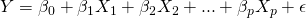where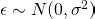, the true value of R squared is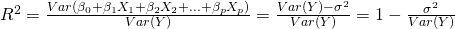The denominator is the total variance of Y, while the numerator is this quantity minus the residual variability not explained by the covariates, i.e. the variability in Y that is explained by the covariates.

Most stats packages present two R squared measures. In R the linear model command gives ‘Multiple R-squared’ and ‘Adjusted R-squared’. What’s the difference, and which should we use? Let’s first look at the ‘Multiple R-squared’. This is an estimate of the population R squared value obtained by dividing the model sum of squares, as an estimate of the variability of the linear predictor, by the total sum of squares: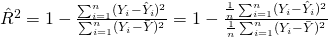where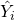denotes the predicted value of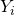and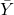denotes the sample mean of Y.

To check this, we can simulate a simple dataset in R as follows:

> set.seed(5132)
> x <- rnorm(10)
> y <- rnorm(10)
> mod <- lm(y ~ x)
> summary(mod)

Call:
lm(formula = y ~ x)

Residuals:
Min      1Q  Median      3Q     Max
-1.2575 -0.7416  0.2801  0.4595  0.9286

Coefficients:
Estimate Std. Error t value Pr(>|t|)
(Intercept)  0.06486    0.28430   0.228    0.825
x           -0.05383    0.25122  -0.214    0.836

Residual standard error: 0.8464 on 8 degrees of freedom
Multiple R-squared:  0.005707,  Adjusted R-squared:  -0.1186
F-statistic: 0.04591 on 1 and 8 DF,  p-value: 0.8357

> anova(mod)
Analysis of Variance Table

Response: y
Df Sum Sq Mean Sq F value Pr(>F)
x          1 0.0329 0.03289  0.0459 0.8357
Residuals  8 5.7310 0.71637
> 0.0329/(0.0329+5.7310)
 0.005707941

This code simulates two vectors X and Y independently, from the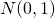distribution. It then fits the linear model for Y with X as covariate. The R-squared value from the summary is 0.005707, suggesting (correctly here) that X is not a good predictor of Y. We then use the anova command to extract the analysis of variance table for the model, and check that the ‘Multiple R-squared’ figure is equal to the ratio of the model to the total sum of squares.

The bias of the standard R squared estimator

Unfortunately, this estimator of R squared is biased. The magnitude of the bias will depend on how many observations are available to fit the model and how many covariates are relative to this sample size. The bias can be particularly large with small sample sizes and a moderate number of covariates. To illustrate this bias, we can perform a small simulation study in R. To do this, we will repeatedly (1000 times) generate data for covariates X, Z1, Z2, Z3, Z4 (independent). We will then generate an outcome Y, which depends only on X, and in such a way that the true R squared is 0.01 (1%). We will then fit the model to each dataset, and record the R-squared estimates:

set.seed(6121)
n <- 20
simulations <- 1000
R2array <- array(0, dim=simulations)
for (i in 1:simulations) {
x <- rnorm(n)
z <- array(rnorm(n*4), dim=c(n,4))
y <- 0.1*x + rnorm(n)
mod <- lm(y~x+z)
R2array[i] <- summary(mod)$r.squared } t.test(R2array) Running this code, I get a mean of the R2array of 0.266, with a 95% CI from 0.257 to 0.274. This compares with the true R squared value of 0.1. Here the simple R squared estimator is severely biased, due to the large number of predictors relative to observations. Why the standard R squared estimator cannot be unbiased Why is the standard estimate (estimator) of R squared biased? One way of seeing why it can’t be unbiased is that by its definition the estimates always lie between 0 and 1. From one perspective this a very appealing property – since the true R squared lies between 0 and 1, having estimates which fall outside this range wouldn’t be nice (this can happen for adjusted R squared). However, suppose the true R squared is 0 – i.e. the covariates are completely independent of the outcome Y. In repeated samples, the R squared estimates will be above 0, and their average will therefore be above 0. Since the bias is the difference between the average of the estimates in repeated samples and the true value (0 here), the simple R squared estimator must be positively biased. Incidentally, lots of estimators we used are not unbiased (more on this in a later post), so lack of unbiasedness doesn’t on its own concern is. The problem is that the bias can be large for certain designs where the number of covariates is large relative to the number of observations used to fit the model. Adjusted R squared So, the simple R squared estimators is upwardly biased. What can we do? Well, we can modify the estimator to try and reduce this bias. A number of approaches have been proposed, but the one usually referred to by ‘adjusted R squared’ is motivated by returning to the definition of the population R squared as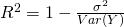The standard R squared estimator uses biased estimators of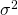and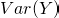, by using the divisor n for both. These estimators are biased because they ignore the degrees of freedom used to estimate the regression coefficients and mean of Y. If instead, we estimateby the usual unbiased sample variance (which uses n-1 as the divisor) andby its unbiased estimator which uses n-p-1 as the divisor, we obtain the estimator: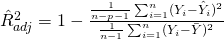where n denotes the sample size, p denotes the number of covariates, and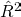denotes the standard R squared estimator. This can be re-arranged to show that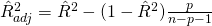From this formula, we can that the adjusted estimator is always less than the standard one. We can also see the larger p is relative to n, the larger the adjustment. Also, as we saw in the simple simulated dataset earlier, it is quite possible for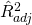to be negative. A final point: although the adjusted R squared estimator uses unbiased estimators of the residual variance and the variance of Y, it is not unbiased. This is because the expectation of a ratio is not generally equal to the ratio of the expectations. To check this, try repeating the earlier simulation, but collecting the adjusted R squared values (stored in summary(mod)$adj.r.squared) and looking at the mean of the estimates.

### 19 thoughts on “R squared and adjusted R squared”

1.hi I have a question. In the Analysis of Variance Table, why the D.F. of x is 1? did you group the values of x into two groups?

•In the setup I simulated X was continuous and entered in the model as a linear covariate. There is one parameter corresponding to X in the model, so the d.f. for it is just 1.

2.In the simulation illustrating the bias of R^2, isn’t the true value equal to 0.01 approximately? Population total variance is 0.1^2*1+1 and population variance explained by the model (due to covariate x) is 0.1^2*1? Isn’t that correct?

•Thank you Giorgos for spotting that! I have corrected it in the post.

3.Hi Jonathan,

How do you test the significance of adjusted R square?

Thank you!

Meng

•Adjusted R square is an alternative estimator of the same parameter as the usual R squared estimator/value. So the global F test for the model and its p value can still be used, even if one prefers to use the adjusted R squared as a point estimate.

•Thanks Jonathan for replying! But are adjusted R square and the usual R square’s estimators/values different from the F test? Since the denominator and degree of freedom for the denominator are different?

•Sorry not sure exactly what you mean. The F-test is a test, with a test statistic, and p-value. Whereas R squared and adjusted R squared are two estimators of the same population parameter. Could you clarify your question?

4.Hello, I was wondering, when I do an F test for the significance of shrinkage on my R^2 the result is significant.
Does that mean the shrinkage of the R^2 is significant or that my model generalizes well to other populations?

5.Your final formula for adjusted R^2 is incorrect. The ratio that is used to “expand” the unexplained variance is (n-1)/(n-p-1), not p/(n-p-1).

•I don’t think so. Try your formula with the first set of simulated data in R. Using the final formula in the post you get what R reports for adjusted R-squared. Using your modification you get something different.

•Apologies! (And apologies for being so slow to respond.) The use of that particular format for the equation is so rare that I didn’t notice the switch from the usual format. My bad.

Out of curiosity, to whom do you believe that that we should give credit for adjusted R^2? Theil (1961)?

6.It says value of r squared lies in the range of 0 – 1. However it should be -∞ to 1.

•I don’t think so. The true R squared must lie between 0 and 1. An estimate of it could be negative, but not the true/population value.

7.Very nice read. But are you sure the adjusted R2 is biased in your example? With 1,000 repetitions, the mean of the adjusted R2 estimates is 0.003488, which seems fairly close to the true population R2 of (approx.) 0.01. Running more simulations, the mean gets very close to theoretical value …

8.Quick note: the block of math markup in the middle of this post (the description of \hat{R^2}) is not displayed properly for me, either on an up-to-date Firefox or on an up-to-date Google Chrome.

•Thanks for alerting me to this. It’s fixed now!

9.•